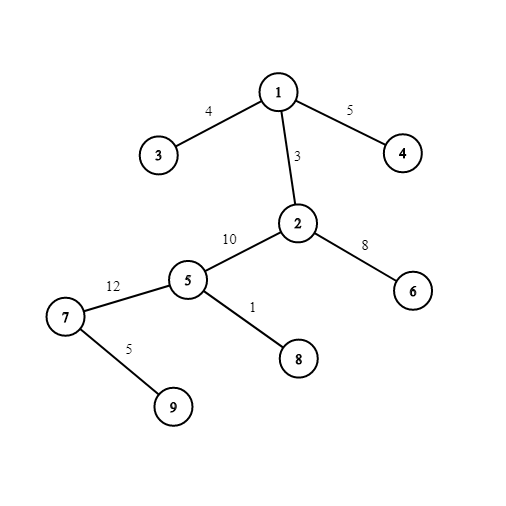Car fuel
Tag(s):

## Algorithms, Binary search algorithm, Euler Tour, Euler Tour and Path, Graph, June Easy 19, Medium-Hard, Segment tree, Tree

Problem
Editorial
Analytics

You are given a weighted tree of $N$ nodes that is rooted at node $1$. The nodes represent the cities in your country. Also, the edges between the nodes $u$ and $v$ represent the fuel that will be utilized while traveling from node $u$ to $v$ or vice versa. Any cars consume the same fuel while moving from node $u$ to $v$ (that is equal to the edge weight between $u$ and $v$). Cars can only move towards the root.

Your task is to answer $Q$ queries of the following type:

In each query, you are provided with two values $u$ and $f$, that denotes that you are currently at node $u$ and your car has $f$ amount of fuel in it initially. You will move from city $p$ to city $q$ only if $q$ is the parent of $p$ and the edge weight between $p$ and $q$ is not more than the fuel left in the car. While moving from node $p$ to node $q$, the fuel in the car decrease by the amount equal to the edge weight between $p$ and $q$. You'll stop whenever you either reach node $1$ or cannot move any further up due to the restriction described above. Also, you cannot pass through a node that has a car parked in it. After you reach your destination, your car will be parked at that node and will affect the subsequent queries. For each query, you are required to print the node where the car is parked.

Note: Assume that no car is parked initially (before query 1).

Input format

• First line: Two space-separated integers $N$ and $Q$ denoting the number of nodes in the tree and the number of queries ($1 \le N, Q \le 10^5$)
• Next $N - 1$ lines: Three space-separated integers $u, v,$ and $w$ representing a road from city $u$ to city $v$ with a weight of $w$ ($1 \le u, v \le N,$ and $1 \le w \le 10^9$)
• Each of the next $Q$ lines: Two space-separated integers $u$ and $f$ denoting the node where you are standing currently and the amount of fuel that your car contain initially ($1 \le u \le N$ and $1 \le f \le 10^{15}$)

Output format

For each query, print the node where the car is parked on a new line.

SAMPLE INPUT
9 6
1 2 3
1 3 4
1 4 5
2 5 10
2 6 8
5 7 12
5 8 1
7 9 5
2 2
5 50
4 4
6 10
7 13
8 500

SAMPLE OUTPUT
2
2
4
2
5
5

ExplanationThe tree is shown in the figure. Initially, no cars are parked.

• In the first query, we are at node $2$ and fuel in the car is $2$ units initially. We cannot move to node $1$ as the edge weight between node $1$ and node $2$ is $3 (>2).$ Hence, the car gets parked at node $2$.
• In the second query, we are at node $5$ and fuel in the car is $50$ units initially. We can move upto node 2 by utilizing $10$ units of fuel. There is already a car parked at node $2$ and thus, we cannot move any further up. Hence, this car also gets parked at node $2$.
• In the third query, we start at node $4$ with initial fuel of $4$ units. As the edge weight is $5$ units between node $1$ and node $4$, we cannot move up and hence, the car gets parked at node $4$.
• In the fourth query, we can move up to node $2$. A car is already parked at node $2$ and hence, we cannot move any further up.
• In the fifth query, we can only move up to node $5$ because we do not have enough fuel to move from node $5$ to node $2$. Thus, the car gets parked at node $5$.
• In the final query, even if we have $500$ units of fuel, we can only move up to node $5$ because it has a car parked already!

Time Limit: 1.0 sec(s) for each input file.
Memory Limit: 256 MB
Source Limit: 1024 KB
Marking Scheme: Marks are awarded when all the testcases pass.
Allowed Languages: Bash, C, C++, C++14, Clojure, C#, D, Erlang, F#, Go, Groovy, Haskell, Java, Java 8, JavaScript(Rhino), JavaScript(Node.js), Julia, Kotlin, Lisp, Lisp (SBCL), Lua, Objective-C, OCaml, Octave, Pascal, Perl, PHP, Python, Python 3, R(RScript), Racket, Ruby, Rust, Scala, Swift, Swift-4.1, TypeScript, Visual Basic

## CODE EDITOR

Initializing Code Editor...

## This Problem was Asked inChallenge Name

June Easy' 19

OTHER PROBLEMS OF THIS CHALLENGE
• Basic Programming > Input/Output
• Algorithms > Sorting
• Algorithms > Greedy Algorithms
• Algorithms > Graphs
• Math > Basic Geometry